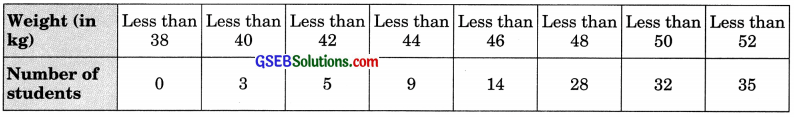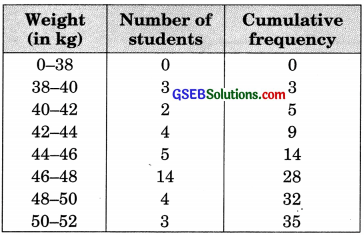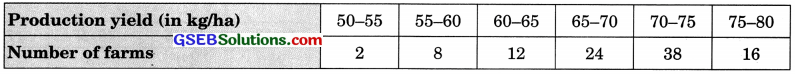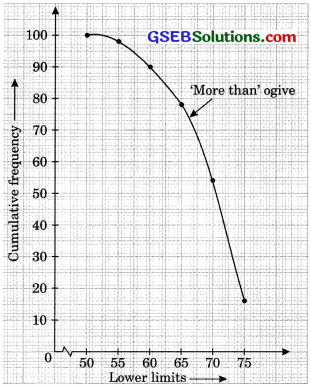# GSEB Solutions Class 10 Maths Chapter 14 Statistics Ex 14.4

Gujarat Board GSEB Solutions Class 10 Maths Chapter 14 Statistics Ex 14.4 Textbook Questions and Answers.

## Gujarat Board Textbook Solutions Class 10 Maths Chapter 14 Statistics Ex 14.4

Question 1.
The following distribution gives the daily income of 50 workers of a factory.Convert the distribution above to a less than type cumulative frequency distribution, and draw it so give.
Solution:
Less than type cumulative frequency distribution.Question 2.
During the medical check-up of 35 students of a class, their weights were recorded as follows:Draw a less than type ogive for the given data. Hence obtain the median weight from the graph and verify the result by using the formula.
Solution:
Here, $$\frac {n}{2}$$ = $$\frac {35}{2}$$ = 17.5
Locate 17.5 on the y-axis. From this point, draw a line parallel to the x-axis cutting the curve at a point. From this point, draw a perpendicular to the x-axis. The point of intersection of this perpendicular with the x-axis determines the median of the given data as 46.5 kg. Median weight by using the formula Grouped Frequency DistributionNow, n = 35
So, $$\frac {n}{2}$$ = $$\frac {35}{2}$$ = 17.5
This observation lies in class 46 – 48.
So, 46 – 48 is the median class.
Therefore, l = 46, h = 2, f= 14, c.f = 14
∴ Median (by using formula)
= 46 + [$$\frac {17.5 – 14}{14}$$ x 2
= 46 + $$\frac {1}{2}$$ = 46.5 kg.
Verification: We find that the median weight obtained from the graph is the same as the median weight obtained by using the formula.Question 3.
The following table gives production yield per hectare of wheat of loo farms of a village.Change the distribution to a more than type distributIon, and draw its ogive.
Solution:More than type of distribution.